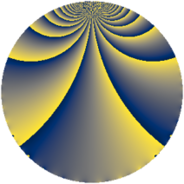# Properties

 Label 1148.2.fLevel $1148$ Weight $2$ Character orbit 1148.f Rep. character $\chi_{1148}(83,\cdot)$ Character field $\Q$ Dimension $160$ Sturm bound $336$

# Learn more about

## Defining parameters

 Level: $$N$$ $$=$$ $$1148 = 2^{2} \cdot 7 \cdot 41$$ Weight: $$k$$ $$=$$ $$2$$ Character orbit: $$[\chi]$$ $$=$$ 1148.f (of order $$2$$ and degree $$1$$) Character conductor: $$\operatorname{cond}(\chi)$$ $$=$$ $$28$$ Character field: $$\Q$$ Sturm bound: $$336$$

## Dimensions

The following table gives the dimensions of various subspaces of $$M_{2}(1148, [\chi])$$.

Total New Old
Modular forms 172 160 12
Cusp forms 164 160 4
Eisenstein series 8 0 8

## Trace form

 $$160q - 2q^{2} + 2q^{4} - 2q^{8} + 160q^{9} + O(q^{10})$$ $$160q - 2q^{2} + 2q^{4} - 2q^{8} + 160q^{9} - 18q^{14} - 6q^{16} - 30q^{18} - 8q^{21} + 8q^{22} - 176q^{25} + 16q^{28} - 16q^{29} + 32q^{30} - 22q^{32} + 10q^{36} - 32q^{37} - 12q^{42} - 16q^{44} - 4q^{46} + 16q^{49} + 50q^{50} + 32q^{53} - 26q^{56} - 32q^{57} - 44q^{58} + 24q^{60} - 10q^{64} + 16q^{65} + 6q^{70} + 48q^{72} + 2q^{74} - 32q^{77} + 66q^{78} + 144q^{81} - 50q^{84} + 48q^{85} + 40q^{86} - 4q^{88} + 22q^{92} - 16q^{93} - 78q^{98} + O(q^{100})$$

## Decomposition of $$S_{2}^{\mathrm{new}}(1148, [\chi])$$ into newform subspaces

The newforms in this space have not yet been added to the LMFDB.

## Decomposition of $$S_{2}^{\mathrm{old}}(1148, [\chi])$$ into lower level spaces

$$S_{2}^{\mathrm{old}}(1148, [\chi]) \cong$$ $$S_{2}^{\mathrm{new}}(28, [\chi])$$$$^{\oplus 2}$$